# NCERT Solutions class-11 Maths Exercise 14.3## myCBSEguide App

CBSE, NCERT, JEE Main, NEET-UG, NDA, Exam Papers, Question Bank, NCERT Solutions, Exemplars, Revision Notes, Free Videos, MCQ Tests & more.

Exercise 14.3

1. For each of the following compound statements first identify the connecting words and then break it into component statements:

(i) All rational numbers are real and all real numbers are not complex.

(ii) Square of an integer is positive or negative.

(iii) The sand heats up quickly in the sun and does not cool down fast at night.

(iv)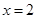and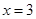are the roots of the equation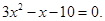Ans. (i) The component statement has the connecting word ‘and’ component statements are: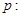All rational numbers are real.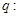All real numbers are not complex.

(ii) The component statement has the connecting word ‘or’ component statements are:Square of an integer is positive.Square of an integer is negative.

(iii) The component statement has the connecting word ‘and’ component statements are:The sand heats up quickly in the sun.The sand does not cool down fast at night.

(iv) The component statement has the connecting word ‘and’ component statements are:

x = 2 is a root of the equation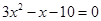y = 3 is a root of the equation2. Identify the quantifier in the following statements and write the negation of the statements:

(i) There exists a number which is equal to its square.

(ii) For every real number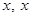is less than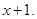(iii) There exists a capital for every state in India.

Ans. (i) Here the qualifier is ‘there exists’.

The negation of statement is: There exists a number which is not equal to its square.

(ii) Here the qualifier is ‘for every’.

The negation of statement is: For at least one real number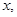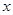is not less than(iii) Here the qualifier is ‘there exists’.

The negation of statement is: There exists a state in India which does not have a capital.

3. Check whether the following pair of statements are negations of each other. Give reasons for your answer.

(i)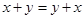is true for every real numbersand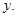(ii) There exists real numbersand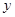for which.

Ans. Letis true for every real numberandAndThere exists real numbersandfor which.

Now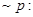There exists real numbersandfor which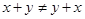therefore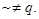4. State whether the ‘or’ used in the following statements is “exclusive” or inclusive”. Give reasons for your answers.

(i) Sun rises or moon sets.

(ii) To apply for a driving license, you should have a ration card or a passport.

(iii) All integers are positive or negative.

Ans. (i) This statement makes use of exclusive ‘or’. Since when sun rises, moon does not set during day-time.

(ii) This statement makes use of inclusive ‘or’. Since you can apply for a driving licence even if you have a ration card as well as passport.

(iii) This statement makes use of exclusive ‘or’. Since all integers are positive as well as negative.